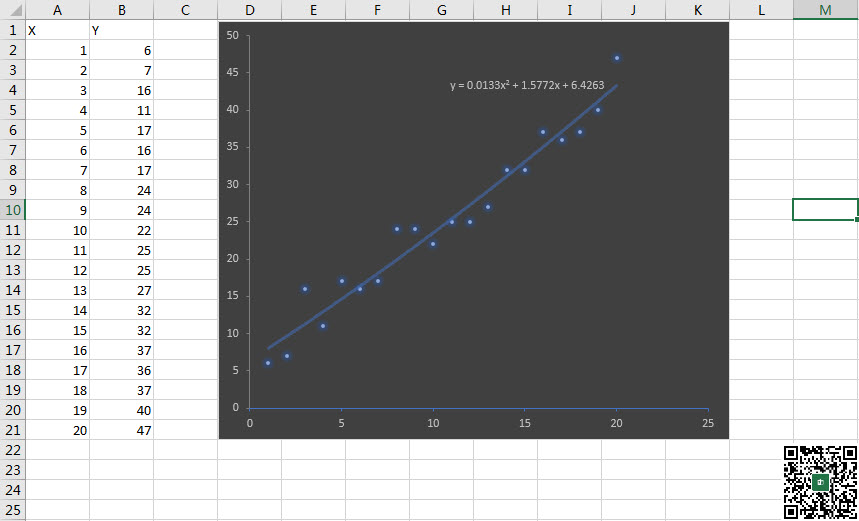﻿ 如何用vba添加图表趋势线、读取趋势线公式以及提取公式中的系数？ - Excel - ExcelOffice【微信公众号：水星Excel】

# 如何用vba添加图表趋势线、读取趋势线公式以及提取公式中的系数？`y = 0.0133x2 + 1.5772x + 6.4263`

```Sub QQ1722187970()
Dim oChart As Chart
Dim oChartObject As ChartObject
Dim oWK As Worksheet
Dim oSeries As Series
Dim oTrendLine As Trendline
Dim oDataLabel As DataLabel
For Each oWK In Excel.ThisWorkbook.Worksheets
Set oChartObject = oWK.ChartObjects(1)
With oChartObject
Set oChart = .Chart
With oChart
For Each oSeries In .SeriesCollection
With oSeries
iCount = 6
Set oTrendLine = .Trendlines.Add(Type:=xlPolynomial, Order:=iCount, DisplayEquation:=True, Name:="TL1", DisplayRSquared:=False)
With oTrendLine
Set oDataLabel = .DataLabel
With oDataLabel
.Select
sFormula = .Text
Debug.Print sFormula
End With
End With
End With
Next
End With
End With
Next
End Sub```